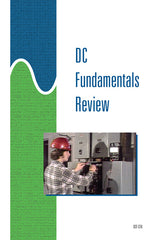#### Get the Study Guide for this videoFollow the video session-by-session with this handy book. Color illustrations throughout. \$15

Instructor Guide  available

# DC Fundamentals Review - Instant Video

##### Summary

This 63-minute video reviews the fundamental relationships between current, voltage, and resistance in DC circuits. It is intended as a review of basic electrical concepts and circuit analysis, for trainees already possessing some background in electrical theory. Specific attention is directed to Ohm’s law, Kirchhoff’s voltage law, Kirchhoff’s current law, and practical techniques for analyzing series circuits, parallel circuits, and series-parallel circuits.

##### Training Sessions
Ohm’s Law – 9 minutes

Names the electrical quantities represented by I, E, and R. States Ohm’s law in words and as a formula. Shows how to calculate the current, voltage, or resistance of a simple circuit when any two of the quantities are known. Presents a memory aid.

Series Circuits – 10 minutes

Describes how to calculate: total resistance of a series circuit when its individual series resistances are known; resistance of a single series component when the total circuit resistance and the remaining series resistances are known. States Kirchhoff’s voltage law, and shows how to calculate the voltage drops across each component in a series circuit.

Parallel Circuits – 12 minutes

States Kirchhoff’s current law. How to calculate the branch currents in a parallel circuit when the source voltage and the individual branch resistances are known, the total current through a parallel circuit when the individual branch currents are known, and the total resistance of a parallel circuit when the individual branch resistances are known.

Series-Parallel Circuits – 12 minutes

Working with resistance strings in parallel, and resistance banks in series. How to calculate: total resistance of a series-parallel circuit, voltage drops in a series-parallel circuit, branch currents in a series-parallel circuit.

Shortcut Methods – 9 minutes

How to use proportional voltage drops, proportional branch currents. Special cases in parallel circuits: two parallel branches, several branches of equal resistance.

Troubleshooting DC Circuits – 11 minutes

Demonstrates how to troubleshoot a DC motor drive circuit, a grounded DC distribution system, a series circuit, a parallel circuit.

Item Code: DCF-IV